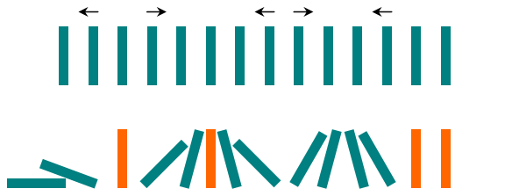# 838. Push Dominoes | LeetCode Solution

## 838. Push Dominoes

There are `n` dominoes in a line, and we place each domino vertically upright. In the beginning, we simultaneously push some of the dominoes either to the left or to the right.

After each second, each domino that is falling to the left pushes the adjacent domino on the left. Similarly, the dominoes falling to the right push their adjacent dominoes standing on the right.

When a vertical domino has dominoes falling on it from both sides, it stays still due to the balance of the forces.

For the purposes of this question, we will consider that a falling domino expends no additional force to a falling or already fallen domino.

You are given a string `dominoes` representing the initial state where:

• `dominoes[i] = 'L'`, if the `ith` domino has been pushed to the left,
• `dominoes[i] = 'R'`, if the `ith` domino has been pushed to the right, and
• `dominoes[i] = '.'`, if the `ith` domino has not been pushed.

Return a string representing the final state.

Example 1:

`Input: dominoes = "RR.L"`
`Output: "RR.L"`
```Explanation: The first domino expends no additional force on the second domino.
```

Example 2:`Input: dominoes = ".L.R...LR..L.."`
```Output: "LL.RR.LLRRLL.."
```

Constraints:

• `n == dominoes.length`
• `1 <= n <= 105`
• `dominoes[i]` is either `'L'``'R'`, or `'.'`.
Explanation:

We will make a deque so that we can push and pop from front and back. For each second we will iterate in the deque and check whether the adjacent dominoes can be affected or not.

If a standing dominoes lie adjacent to a domino pushed left then it will get affected and it will fall on the left side.

If a standing domino lies right to a domino pushed right then we need to check two conditions. As it can still stand straight if the condition is

R . L

If there is no Left falling domino then it will be pushed toward the right.

We also need to take care of pushing the new falling dominoes.

Check the Code below for a better understanding.